# TOC | Designing Finite Automata from Regular Expression (Set 4)

In the below article, we shall see some Designing of Finite Automata form the given Regular Expression-

Regular Expression 1: Regular language,

`L1 = (a+b)(a+b) `

The language of the given RE is,

`{aa, ab, ba, bb} `

Length of string exactly 2.

Its finite automata will be like below-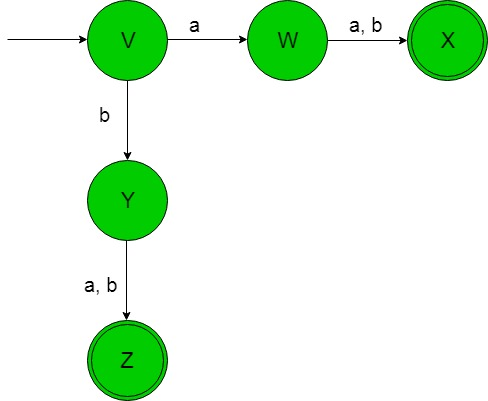In the above transition diagram, as we can see that state ‘V’ on getting ‘a’ as the input it transits to a state ‘W’ which on getting either ‘a’ or ‘b’ as the input it transits to a final state ‘X’ and so on for the remaining states. Thus this FA accepting all the strings of the given RE language.

Regular Expression 2: Regular language,

`L2 = (a+b)(a+b)(a+b)* `

The language of the given RE is,

`{aa, ab, ba, bb, aaa, aab, .........} `

Length of string at least 2.

Its finite automata will be like below-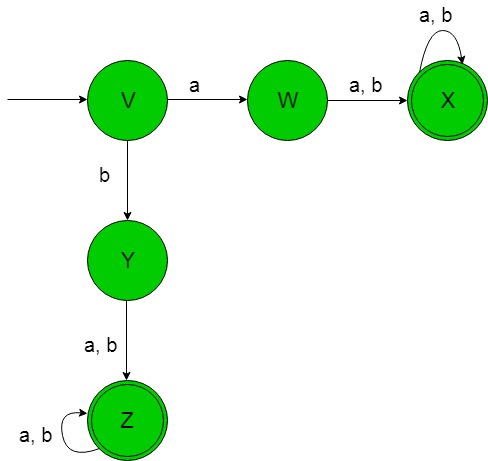In the above transition diagram, as we can see that state ‘V’ on getting ‘a’ as the input it transits to a state ‘W’ which on getting either ‘a’ or ‘b’ as the input it transits to a final state ‘X’ which on getting either ‘a’ or ‘b’ it remains in the state of itself and so on for the remaining states. Thus this FA accepting all the strings of the given RE language.

Regular Expression 3: Regular language,

`L3 = (a+b+ε)(a+b+ε) `

The language of the given RE is,

`{ε, a, b, aa, ab, ba, bb} `

Length of string at most 2.

Its finite automata will be like below-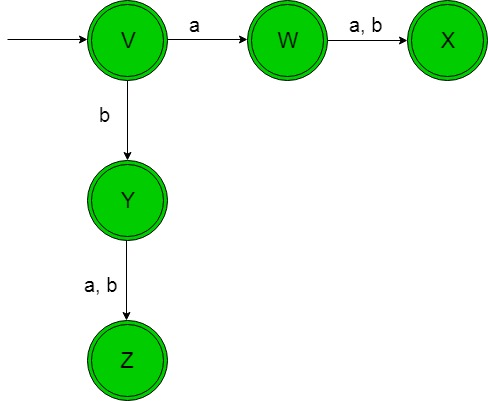In the above transition diagram, as we see that an initial and final state ‘V’ on getting ‘a’ as the input it transits to another final state ‘W’ which on getting either ‘a’ or ‘b’ as the input it transits to another final sate ‘X’ and so on for the remaining states as well . Thus this FA accepting all the strings of the given RE language.

Regular Expression 4: Regular language,

`L4 = ((a+b)(a+b))* `

The language of the given RE is,

`L4 = {ε, aa, ab, ba, bb, aaaa, ............} `

Language of even length string.

Its finite automata will be like below-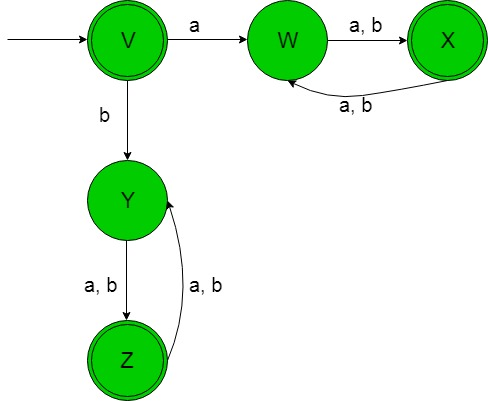In the above transition diagram, the initial and final state ‘V’ on getting ‘a’ as input it goes to a state ‘W’ which on getting either ‘a’ or ‘b’ as the input it transits to another final state ‘X’ which on getting either ‘a’ or ‘b’ as the input it goes back to the state ‘W’ and so on for the remaining states as well. Thus this FA accepting all the strings of the given RE language.

Regular Expression 5: Regular language,

`L5 = ((a+b)(a+b))*(a+b) `

The language of the given RE is

`{a, b, aaa, bbb, abb, bab, bba, ..........} `

Language of odd length string.

Its finite automata will be like below-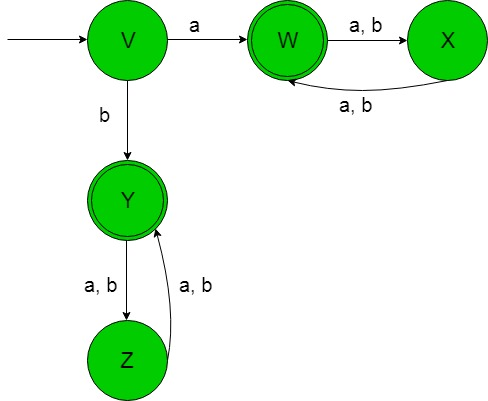In the above transition diagram, the initial state ‘V’ on getting ‘a’ as input it goes to a final state ‘W’ which on getting either ‘a’ or ‘b’ as the input it transits to another state ‘X’ which on getting either ‘a’ or ‘b’ as the input it goes back to the final state ‘W’ and so on for the remaining states as well. Thus this FA accepting all the strings of the given RE language.My Personal Notes arrow_drop_upCheck out this Author's contributed articles.

If you like GeeksforGeeks and would like to contribute, you can also write an article using contribute.geeksforgeeks.org or mail your article to contribute@geeksforgeeks.org. See your article appearing on the GeeksforGeeks main page and help other Geeks.

Please Improve this article if you find anything incorrect by clicking on the "Improve Article" button below.

Article Tags :

Be the First to upvote.

Please write to us at contribute@geeksforgeeks.org to report any issue with the above content.